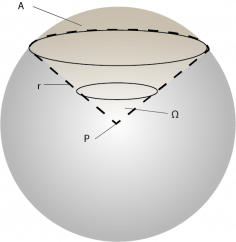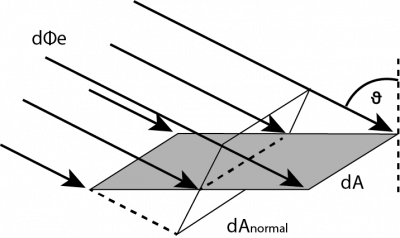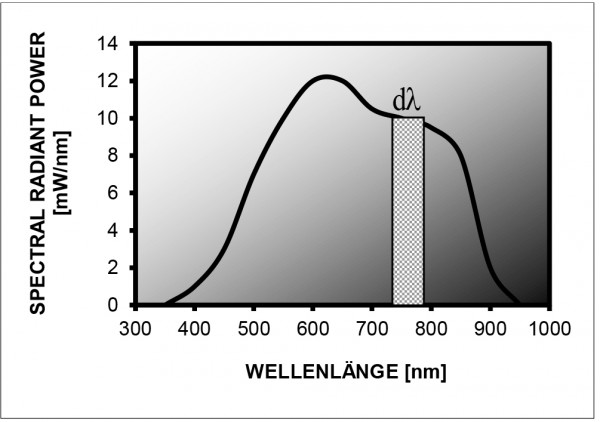#### Quick Contact

Phone: +49 (0)8193-93700-0
info@gigahertz-optik.de

Gigahertz-Optik, Inc. (US office)
Phone: +1-978-462-1818
info-us@gigahertz-optik.com

#### Watchlist

You can add products to the watchlist and compare them with one another or send us an inquiry. There are watchlist symbols on product pages and product tables for this purpose.

The whole discipline of optical measurement techniques can be roughly subdivided into photometry and radiometry. Whereas photometry focuses on determining optical quantities that are closely related to the sensitivity of the human eye, radiometry deals with the measurement of energy per time (= power, given in watts) emitted by light sources or impinging on a particular surface. Thus, the units of all radiometric quantities are based on watts (W). According to CIE regulations, symbols for radiometric quantities are denoted with the subscript “e” for “energy”. Similarly, radiometric quantities given as a function of wavelength are labelled with the prefix “spectral” and the subscript “λ” (for example spectral radiant power Φλ).

Remark: The definitions of radiometric quantities cannot be understood without a basic comprehension of differential quantities. For an intuitive understanding of these quantities, which is the main objective of this paragraph, the differential quantities dλ, dA and dΩ can be regarded as tiny intervals or elements (ΔλΔA and ΔΩ) of the respective quantity. As a consequence of the fact that these intervals or elements are very small, radiometric quantities can be considered constant over the range defined by dλ, dA and / or dΩ. Similarly, dΦe, dIe, dLe and dEcan be regarded as small portions which add up to the total value of the respective quantity. Find here an brief explanation of the concept of differential quantities and integral calculus for spectral radiometric quantities.

## Definition of solid angle

The geometric quantity of a solid angle Ω quantifies a part of an observer’s visual field. If we imagine an observer located at point P, his full visual field can be described by a sphere of an arbitrary radius r (see fig. 1). Here, a certain part of this full visual field defines an area A on the sphere’s surface and the solid angle Ω is defined as

 Ω = A r²

Since the area A is proportional to r², this fraction is independent of the actual choice of r.

If we want to calculate the solid angle determined by a cone (as shown in fig. 1) area A is the area of a spherical calotte. However, area A can have any arbitrary shape on the sphere’s surface because the solid angle is only defined for conical parts of the full visual field.

Although Ω is dimensionless, it is common to use the unit steradian (sr). The observer’s total visual field is described by the whole surface of the sphere, which is given by 4πr², and thus covers the solid angle

 Ωtotal = 4π r² = 4π sr = 12.57 sr r²Fig. 1: The solid angle Ω quantifies a certain part of the visual field seen by an observer located at P

Source (valid as of 2002): http://whatis.techtarget.com/definition/0,,sid9_gci528813,00.html

Radiant power Φe is defined as the total power of radiation emitted by a source (lamp, light emitting diode, etc.), transmitted through a surface, or impinging upon a surface. Radiant power is measured in watts (W). The definitions of all other radiometric quantities are based on radiant power. If a light source emits uniformly in all directions, it is called an isotropic light source.

Radiant power characterizes the output of a source of electromagnetic radiation only by a single number and does not contain any information on the spectral distribution or the directional distribution of the lamp output.Fig. 2: The radiant power (Φe) of a light source is given by its total emitted radiation

Radiant intensity Ie describes the radiant power of a source emitted in a certain direction. The source’s (differential) radiant power e emitted in the direction of the (differential) solid angle element dΩ is given by

dΦ= Ie dΩ

and thus

 Φe = ∫ Ie dΩ 4π

In general, radiant intensity depends on spatial direction. The unit of radiant intensity is W / sr.Fig. 3: Typical directional distribution of radiant intensity for an incandescent bulb

The radiance Le is the intensity of optical radiation emitted or reflected from a certain location on an emitting or reflecting surface in a particular direction (the CIE definition of radiance is more general. This tutorial focuses on the most relevant radiance application describing the spatial emission characteristics of a source). The radiant power dΦe emitted by a (differential) surface element dA in the direction of the (differential) solid angle element dΩ is given by

Equation 1:
e = Le × cos(ϑ) dA dΩ

In this relation, ϑ is the angle between the direction of the solid angle element dΩ and the normal of the emitting or reflecting surface element dA.

From the definition of radiant intensity Ie, it follows that the differential radiant intensity emitted by the differential area element dA in a certain direction is given by

dIe = Le cos(ϑ) dA

Thus,

Equation 2:

 Ie = ∫ Le × cos(ϑ) dA emitting surface

whereby ϑ is the angle between the emitting surface element dA and the direction for which Ie is calculated.

The unit of radiance is W/(m2sr).

The irradiance Ee is the amount of radiant power impinging upon a surface per unit area. In detail, the (differential) radiant power dΦe upon the (differential) surface element dA is given by

= Ee dA

Generally, the surface element can be oriented at any angle towards the direction of the beam. However, irradiance is maximized when the surface element is perpendicular to the beam:

= Ee,normal dAnormalFig. 4: Irradiance is defined as incident radiant power dΦe per surface area element dA

Note that the corresponding area element dAnormal, which is oriented perpendicular to the incident beam, is given by

dAnormal = cos(ϑ) dA

with ϑ denoting the angle between the beam and the normal of dA, we get

Equation 3:
E= Ee,normal × cos(ϑ)

The unit of irradiance is W/m2.

Radiant exitance Me quantifies the radiant power that is emitted or reflected from a certain location on a surface per area. In detail, the (differential) radiant power dΦe emitted or reflected by the surface element dA is given by

e = MdA

Based on the definition of radiance, the (differential) radiant exitance dMe emitted or reflected by a certain location on a surface in the direction of the (differential) solid angle element dΩ is therefore given by

dMe = Le cos(ϑ) dΩ

and consequently

Equation 4:

 Me = ∫ Le × cos(ϑ) dΩ 2πsr

The integration is performed over the solid angle of 2π steradian corresponding to the directions on one side of the surface and ϑ denotes the angle between the respective direction and the surface’s normal.

The unit of radiant exitance is W/m². In some particular cases, Me = Ee (see  “Reflectance ρ, Transmittance τ and Absorptance α” ).

The radiometric quantities discussed above are defined without any regard to the wavelength(s) of the quantified optical radiation. In order to not only quantify the absolute amount of these quantities but also the contribution of light from different wavelengths, it is important to also define the respective spectral quantities.

Spectral radiant power is defined as a source’s radiant power per wavelength interval as a function of wavelength. In detail, the source’s (differential) radiant power e emitted in the (differential) wavelength interval between λ and λ+dλ is given by

dΦe = Φ λ (λ) dλ

This equation can be visualized geometrically (see Fig. 5). Because dλ is infinitesimally small, spectral radiant power Φλ(λ) is approximately constant in the interval between λ and λ+dλ. Thus, the product Φλ(λ)dλ equals the area under
the graph of Φλ(λ) in the interval between λ and λ+dλ. This area describes the contribution of this very wavelength interval to the total value of radiant power Φe, which is graphically represented by the total area under the graph of spectral radiant power Φλ(λ).

Mathematically, this can be expressed by the integral

 Φe = ∞ ∫ Φ λ(λ) dλ 0

The unit of spectral radiant power is W/nm or W/Å.

The other spectral quantities are defined correspondingly and their units are given by the unit of the respective quantity, divided by nm or Å. Generally, a radiant quantity can be calculated from the respective spectral quantity by integrating over the wavelength from λ = 0 to λ = ∞. However, this integration is often restricted to a certain wavelength range, which is indicated by the respective prefix. For instance, UV-A irradiance is defined as

 Ee,UV-A = 400 nm ∫ Eλ(λ) d 315 nm

since the UVA range is between λ = 315 nm and λ = 400 nm.Fig. 5: Relation between spectral radiant power Φλ(λ) and radiant power Φe, visualized  at a hypothetical example.
Radiant power emitted in the wavelength interval between λ  and λ+dλ  is given by the area of the shaded rectangle,
which amounts to Φλ(λ)dλ
The total amount of radiant power Φe emitted over  the whole spectrum is given
by the area under
the curve describing Φλ(λ), which is mathematically expressed by an integral.

## Integral measuring Devices

Measuring instruments that directly provide the integrated, i.e. not spectrally resolved, radiometric unit are often called integral measuring devices. These in turn are specially optimized for the various applications in their spectral sensitivity as well as design to ideally meet the applications.

## Spectral measuring Devices

Measuring instruments which output the optical radiation spectrally resolved and in radiometric units are called spectral measuring devices or spectroradiometers. These Spectroradiometers are also optimally adapted to the application in their design and specifications.